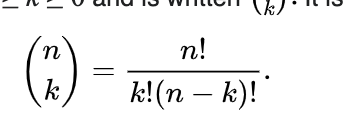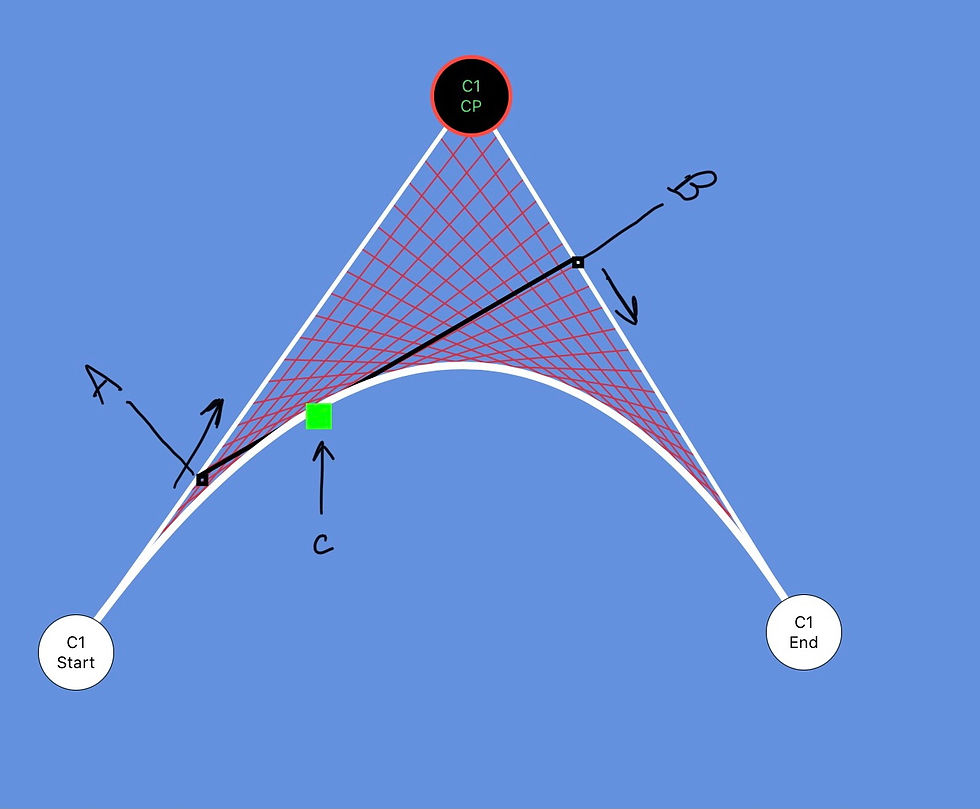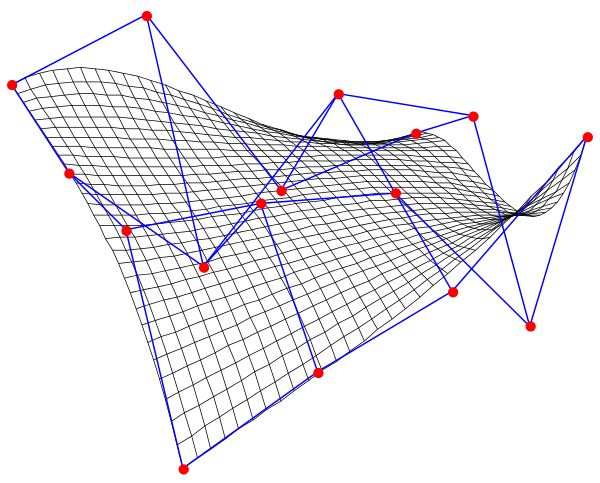top of page

# A Deep Dive into Bézier Curves## What are Bézier Curves?

This article will begin with a brief history of how Bezier Curves’ came to be and then transition into a deep dive into the mathematics powering the bezier curve equation.

The mathematics following the history of bezier curves can be somewhat dense, so if you find the beginning boring, skip to the geometric interpretation or animation application section towards the end of the article.

These sections are less math-intensive and more visual-based, but still, give a great representation of what the mathematics behind Bezier Curves ends up creating.

Finally, to learn even more, check out my iOS app: Bezier Playground.

My app is a bezier curve generator that allows you to manipulate Bezier curves on a canvas and change the animation speed along with frame rate, all in real-time.

The app also provides some interactive examples to provide a more interactive learning experience.

## History of Bézier Curves

The development of Bézier curves represents a critical point in Computer-aided design history.

Bezier curves assist with the process of drawing smooth curves. These curves benefit many industries ranging from graphic design to automobile design, where they first were popularized.

Bezier curves commemorate Pierre Bézier, an engineer who, while working at Renault in the 1960s, developed a method to describe parametric surfaces as a mathematical polynomial.

Many sources view this as the beginning of computer-aided design technology because engineers could now define complex smooth shapes with math, rather than having to design them by hand.

These curves had an immediate effect on the business. In1968 the entire body of the Peugeot model 204 was designed via the CAD software UNISURF as shown below:Bezier curves continued to gain traction over the next 20 years in the CAD industry, eventually resulting in Pierre Bezier receiving a Steven A. Coons award from ACM SIGGRAPH for his lifetime contribution to computer graphics and interactive techniques.”

However, the history of Bezier curves is much deeper than Pierre Bezier himself. There is a rich history of mathematicians before him who had to make immense leaps to make bezier curves possible—the first being Sergei Natanovich Bernstein with the invention of the Bernstein polynomial.

## Bernstein Polynomials

a Bernstein polynomial is a polynomial that is a linear combination of Bernstein basis polynomials.

### Weierstrass approximation theorem.• I won’t cover the theorem in detail, but it states: “that every continuous function defined on a closed interval [a, b] can be uniformly approximated as closely as desired by a polynomial function.”. This is shown in the figure above.

• Furthermore, “Because polynomials are among the simplest functions, and because computers can directly evaluate polynomials, this theorem has both practical and theoretical relevance, especially in polynomial interpolation.

Now that we have a general idea of what Berstein Polynomials are generally, I will dive into the math behind them.

### Introducing the math behind Bernstein basis polynomials :The above can look complicated, but it is just an application of the binomial theorem & the binomial coefficient.• (n /v) should be read as "n choose "v" and is the binomial coefficient

### Binomial Theorem

We must first understand the binomial theorem in order to understand the binomial coefficient which states:

“It is possible to expand the polynomial (x + y)^n into a sum involving terms of the form a*x^b*y^c, where the exponents b and c are nonnegative integers with b + c = n, and the coefficient a of each term is a specific positive integer depending on n and b.

Once again, this definition is somewhat complicated, so it is easier to show via an example below.

### Binomial Theorem Example (n=4)• As you can see the binomial theorem allows us to expand our original expression into a sum of terms.

• The coefficient that occurs in front of each of these terms is the binomial coefficient. (In the above example these terms are 1,4,6,4,1 in that order).

### Defining Binomial Coefficient• The binomial coefficient allows us to determine the coefficient associated with each term in our sum of terms when using the binomial theorem. This is formally described in the expression above.

• However, there is a more efficient way to compute the binomial coefficient as the above factorial definition is very computationally intensive.

• We can leverage Pascal’s triangle to quickly determine the coefficient.

• When using Pascal's triangle it is important to remember, we read the binomial coefficient as “n choose v”.

• In Pascal's triangle, n is the row, and v is the column in the triangle resulting in the coefficient.

• Finally, when looking for the row and column is it important to remember the index for both starts at zero in Pascal's triangle

### Pascals Triangle• For the example n =4, we can see that “4 choose 0” results in “1” which is why for our first term (x⁴) the coefficient is “1”

• For the next term, “4 choose 1” we can see the coefficient is “4” which corresponds to the 4th row and 1st indexed element in the triangle.

### Applying Binomial coefficient to a Bernstein polynomial• The above is an example of the binomial coefficient when applied to a Bernstein polynomial

• The above should be read as “5 choose 2” which allows us to procure our coefficient of “10”, by going to row 5, column 2, of Pascal’s triangle.

The binomial theorem, binomial coefficient, and pascals triangle have a rich history and many applications alone that I might one day write separate articles on, but to mathematically understand Bernstein polynomials, and in turn bezier curves, all that is needed is a basic understanding of what they are.

### Revisiting the math behind Bernstein basis polynomials :• The above formula should make a lot more sense now that we understand the binomial coefficient.

• We determine the coefficient in front of the expression x^v(1-x)^(n-v) via the binomial coefficient

• Finally, determining the exponents of x & 1-x is simply a matter of following the formula based on v and n-v. (n is the degree, v is the current iteration).

## Berstein basis polynomial examples• As you can see each exponent of (1-x) corresponds to the result of n-v, and each exponent of “x” corresponds to “v” and finally, each coefficient corresponds to the binomial coefficient, which once again we can look up in Pascals Triangle using “n choose v”.

• Now admittedly, these Bernstein basis polynomials are not that impressive by themselves, kinda boring, and even a headache to figure out & understand.

• However, they are the fundamental mathematical basis of bezier curves, have been the foundation for many other mathematical innovations, and without these polynomials, the curves would not be possible.

Berstein polynomials were established in 1912, but it wasn’t until 1959, with the invention of de Casteljau’s algorithm, and then in the 1960s with the formalization of Bezier curves that these polynomials found a real-world application.

## de Casteljau’s algorithm

Paul de Casteljau, a highly gifted mathematician, devised a system based on the use of Bernstein polynomials. … the system devised by de Casteljau was oriented towards translating already existing shapes into patches, defined in terms of numerical data"
“In the mathematical field of numerical analysis, De Casteljau’s algorithm is a recursive method to evaluate polynomials in Bernstein form or Bézier curves

### Bezier Curve Mathematical Definition (as De Casteljau algorthim)• The above describes a Bezier curve (De Casteljau technically defined them before Pierre Bezier formalized them & patented them).

### Revisiting Berstein basis polynomial in Bezier Form• “bi,n(t)” is the Berstein basis polynomial that we just learned about.

• The only differences are that we replace our “x” variable with “t” to more explicitly represent time (more on that later).

• We also change “k” to “i” to more explicitly representing that “i” is a result of a summation or to represent an “index”.

### Bezier Curve Construction

We finally have all the pieces necessary to construct our Bezier Curve.• The above equation is essentially the same as De Casteljau’s algorithm, except we use the variable Pi to explicitly state that it will be a control point on the bezier curve.

• We also restrict t to the interval [0,1].

• The equation described above allows us to construct a smooth curve from an infinite amount of control points, by taking the summation of those points multiplied by the Bernstein polynomial from 0->n as described by the equation above.

• This is very powerful as we now have a mathematical formula to create smooth curves, and is essential for creating realistic and reliable models in CAD programs — This is a huge milestone for CAD.

## Quadratic Bezier Curve Formula Example• The above is an example of a Quadratic Bezier Curve (3 control points) from my app Bezier Playground• We procure the formula for our curve via Berstein Polynomials & deCasteljau’s algorithm

• The result for when n=3 and applied to deCasteljau’s algorithim is described above

• The actual bezier curve is traced out in white, and you can see a green cube moving along the curve

• The green cube moving from C1Start to C1End is a representation of “t” increasing from 0->1.

• As t increases, the calculation is performed, and the Bezier curve is traced out.

### Geometric Interpretation

Now that we understand mathematically how deCasteljau’s algorithm works, I think the cooler part is the geometric result of the math.

The algorithm calculates a point between each of the control points dependent on t in a recursive fashion• First, it calculates a point between C1 start and CP which we will call A

• Next, a point between CP and C1End we will call B.

• After calculating A & B, it calculates a point between A & B that we will call C

• C is the point that actually represents the Bezier Curve.

• C increase between A&B as t increases from 0->1 and the result of C's path will be the bezier curve being traced out between C1Start and C1 end as shown above

• When t =0, A and B would be at equal their respective start points (C1 start &CP)

• Currently t =0.25 so they are 25% down their respective lines away from their respective start points• When T = 0.5 A and B are exactly in between their start and endpoints, and C is exactly between C1Start and C1End as shown above.• When T =0.75 they are 25% away from their respective endpoints and 75% away from their respective start points as shown above.

### Real-Time Calculation Example

• The above video shows the calculation I just described occurring in my app Bezier Playground.

• In this example t is increasing from 0->1 over 5 seconds, resulting in the 5-second animation above.

As you can see, the geometric interpretation is fairly straightforward and easy to understand. This is why Bezier curves are used in so many graphic design tools in addition to CAD programs, designers are easily able to manipulate control points, and the result is the traced out curve is manipulated as well. The next example shows this manipulation in real-time.

### Manipulating the curve

• The above example further illustrates how the bezier curve is a parametric function of its control points.

• The fact that the curve is mathematically defined by its control points allows it to be manipulated in the way shown above.

### Animation Application

• Bezier curves are also very popular in animation software as animators are able to manipulate the curve in order to create different animation effects.

• For example, a straight line will just animate linearly at a constant speed, however, we can manipulate Bezier curves to have animations start somewhat fast, slows down, and then speeds up towards the animation.

• There are standard animation curves are ease-in, ease-out, and ease-in/ease-out.

• You are able to create any of these types of curves at any speed or frame rate in my app.

• However, below are recordings of the various standard animation curves so you can have a better idea before you create your own.

#### Ease-in/Ease-out.

• This last curve to form the Ease-In/Ease-Out animation has 4 control points, this is an example of a cubic bezier curve.

• One of the most powerful things about bezier curves is that they are able to be expanded to an infinite number of control points!

### Expanding the Geometric Interpretation from 3 points to 4 points

So a quadratic bezier curve is pretty cool and has a lot of applications, but the powerful part about the mathematics behind the bezier curve is that it can be expanded to an infinite amount of control points.

This is because the algorithm is defined recursively as a function of n, where n is equal to the number of control points in the curve, this allows us to create an infinite amount of complex curves.• First, we calculate points between each of the control points dependent on t. ( A point between C1Start & CP1 we will call A, a point between CP1 and CP2 we will call B and a point between CP2 and C1End we will call C.

• When t =0, A, B, C = their respective start points (C1Start, CP1, and CP2) and when t =1 they = their respective endpoints (CP1, CP2, and C1End) when t = 0.5 they are exactly in between their start and endpoints as shown above.

• Next, it calculates a point between A & B that we will call D, and a point between B & C we will call E. These points increase between A&B & B&C respectively as t increases from 0->1.

• Finally, it calculates a point between D & E that we will call F, this point is the point that actually represents the Bezier Curve. F will increase between D&E as t increases from 0->1 and the result will be the bezier curve being traced out as shown in the image above.

### 6 Control point Bezier Example:• As stated before, we can construct bezier curves up to an infinite amount of control points.

• However, It becomes more computationally expensive to compute the curves as control points increase, so quadratic and cubic bezier curves are the most popular.

### More complex curves: Composite Bezier Curves, B-Spline’s, and Bezier Surfaces• In addition to the Bezier curves I described above, we are also able to form composite Bezier Curves & B-Splines by attaching two bezier curves of any degree together

• We are also able to expand Bezier Curves from 2-dimensions to 3-dimension by defining Bezier Surfaces, and in turn, can describe composite bezier surfaces as well.

• This article just scratches the surface of all the possibilities with Bezier Curves.

• Perhaps in the future, I will cover composite Bezier Curves, B-Splines, and Bezier Surfaces in more detail.